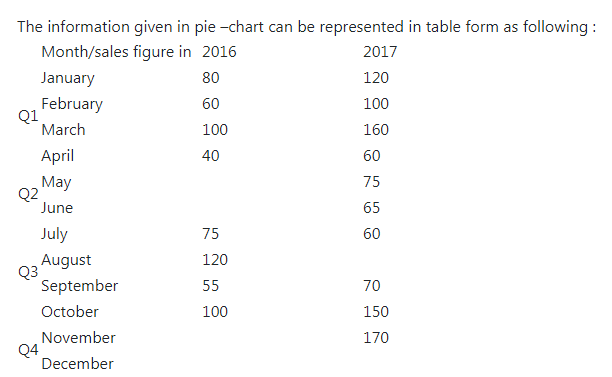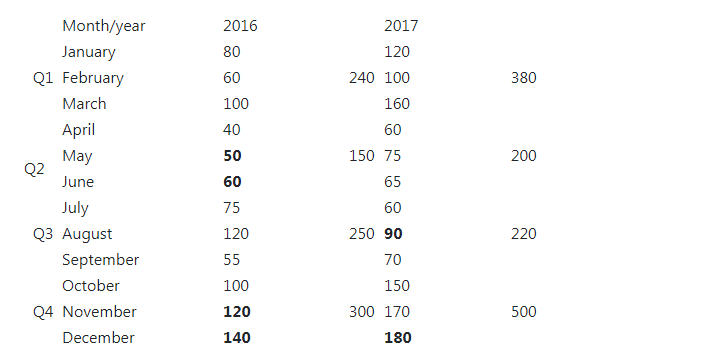# The multi-layered pie-chart below shows the sales of LED television sets |CAT DILR Questions - LRDI | Logical Reasoning |

### Since 2015, the CAT DILR section has become increasingly difficult. In order to tackle the tougher CAT Level DILR questions for the CAT Exam, it is important to understand the basics of CAT. Data Interpretation sets and Logical reasoning puzzles were distinct in DILR. Data Interpretation and Logical Reasoning used to be about computation and understanding charts, graphs, and tables. Within that one hour, you get 5 high-quality puzzles, with some of them being quite challenging. You will solve 30 actual CAT puzzles in CAT 2018 and CAT 2019. The purpose of this page is to help you prepare for that. Take a crack at those puzzles!To obtain a great CAT score, make use of MBAP Free Study material with detailed solutions and video explanations. Check out MBAP free Mock test to take these questions in a test format for free.

You may also find remaining question solution of CAT 2018, slot 1 by searching the question in the search bar.

## CAT 2018 – Slot 1 - Logical Reasoning - Set 1 - The multi-layered pie-chart below shows the sales of LED television sets

### Since 2015, the CAT DILR section has become increasingly difficult. In order to tackle the tougher CAT Level DILR questions for the CAT Exam, it is important to understand the basics of CAT. Data Interpretation sets and Logical reasoning puzzles were distinct in DILR. Data Interpretation and Logical Reasoning used to be about computation and understanding charts, graphs, and tables. Within that one hour, you get 5 high-quality puzzles, with some of them being quite challenging. You will solve 30 actual CAT puzzles in CAT 2018 and CAT 2019. The purpose of this page is to help you prepare for that. Take a crack at those puzzles! To obtain a great CAT score, make use of MBAP Free Study material with detailed solutions and video explanations. Check out MBAP free Mock test to take these questions in a test format for free. You may also find remaining question solution of CAT 2018, slot 1 by searching the question in the search bar. The question below is from previous year CAT question from CAT 2018 exam comes from sub-section Logical Reasoning: 1600 satellites were sent up by a country ... Find out by answering this question which tests an aspirant’s DILR Ability Skills:

Q. 1: What is the percentage increase in sales in December 2017 as compared to the sales in December 2016?1. 28.57
2. 22.22
3. 50.00
4. 38.46

##### Q. 2: In which quarter of 2017 was the percentage increase in sales from the same quarter of 2016 the highest?1. Q12. Q33. Q44. Q2

Q. 3: During which quarter was the percentage decrease in sales from the previous quarter’s sales the highest?
1. Q2 of 2017
2. Q1 of 2017
3. Q4 of 2017
4. Q2 of 2016

Q. 4:During which month was the percentage increase in sales from the previous month’s sales the highest?
1. March of 2017
2. October of 2017
3. October of 2016
4. March of 2016Now as given Sales figure in 2017 Q4 = 500

So sales figure in December 2017 = 500 – 150 – 170 = 180

Total sales in 2017 Q3 = 220

So sales in August 2017 = 220 – 60 – 70 = 90

Similarly sales in missing month of 2016 can be obtained as sales in the three months of 2016 Q4 & Q2 are in Arithmetic Progression. Thus following table can be obtained –Question 1) required percentage increase = (180 – 140)/140×100 =40/140×100=28.57%

Correct option d) 28.57 %

Question 2) it can be seen that the required percentage increase in sales is highest for Q4 and this is = ((500-300))/300×100=66.66%

Correct option a) Q4

Question 3) From the table obtained , we can see there is decrease only in Q2 of both year

Decrease in Q2- 2016 = (240-150)/240×100=37.5%

Decrease in Q2- 2017 = (380-200)/380×100=47.37%

Correct option b) Q2 of 2017

Question 4) As given options are only for may and October and we can see that sales in October 2017 is more than double ( from 70 in November to 150 in October ) so it is highest than other given month .

Correct option a) October 2017

### Past Year Question Paper & SolutionsCounselling Session
By IIM Mentor

### Free Material Area